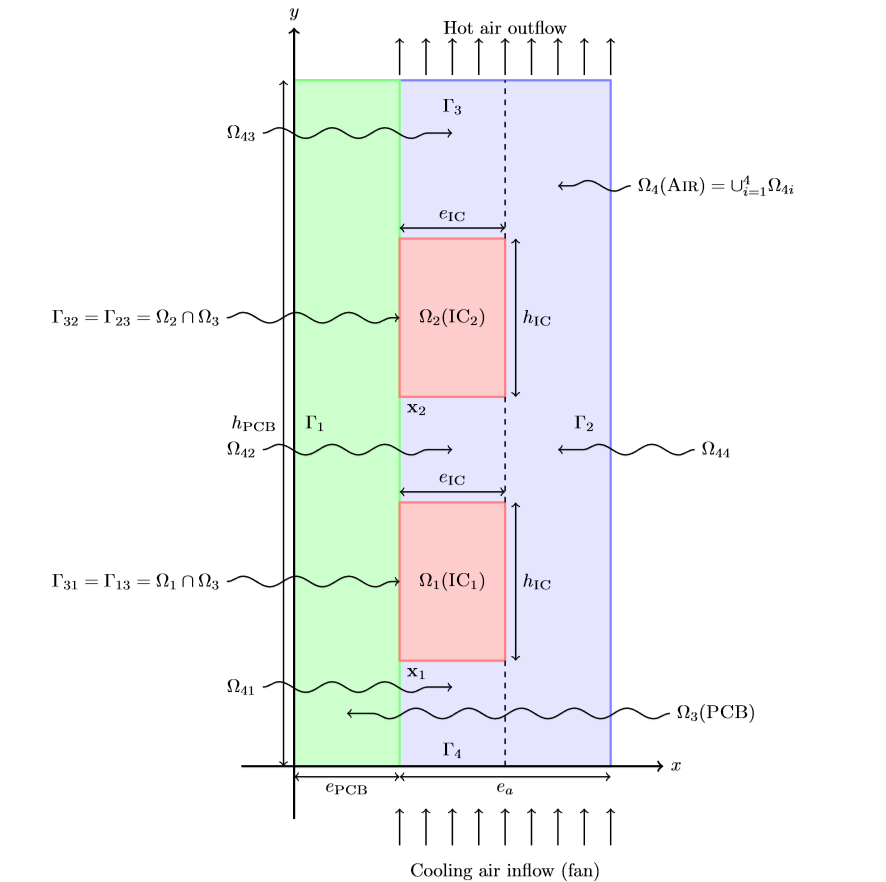# Opus Heat

This test case has been proposed by Annabelle Le-Hyaric and Michel Fouquembergh formerly at AIRBUS.

We consider a 2D model representative of the neighboring of an electronic component submitted to a cooling air flow. It is described by four geometrical domains in $\mathbb{R}^2$ named $\Omega_i,i=1,2,3,4$, see figure. We suppose the velocity $\vec{v}$ is known in each domain --- for instance in $\Omega_4$ it is the solution of previous Navier-Stokes computations. --- The temperature $T$ of the domain $\Omega = \cup_{i=1}^4 \Omega_i$ is then solution of heat transfer equation :

$\begin{equation} \label{eq:1} \rho C_i \Big( \frac{\partial T}{\partial t} + \vec{v} \cdot \nabla T \Big) - \nabla \cdot \left( k_i \nabla T \right) = Q_i,\quad i=1,2,3,4 \end{equation}$

where $t$ is the time and in each sub-domain $\Omega_i$, $\rho C_i$ is the volumic thermal capacity, $k_i$ is the thermal conductivity. $k_1$ and $k_2$ are parameters of the model.

ICs dissipate heat, so the volumic heat dissipated $Q_1$ and $Q_2$ are also parameters of the model, while $Q_3=Q_4=0$.One should notice that the convection term in heat transfer equation may lead to spatial oscillations which can be overcome by Petrov-Galerkin type or continuous interior penalty stabilization techniques.

Integrated circuits (ICs) (domains $\Omega_1$ and $\Omega_2$ ) are respectively soldered on PCB at $\mathbf{x1}=(e_{Pcb}, h_1)$ and $\mathbf{x_2}=(e_{Pcb}, h_2)$. They are considered as rectangles with width $e_{IC}$ and height $h_{IC}$. The printed circuit board (PCB) is a rectangle $\Omega_3$ of width $e_{PCB}$ and height $h_{PCB}$. The air(Air) is flowing along the PCB in domain $\Omega_4$. Speed in the air channel $\Omega_4$ is supposed to have a parabolic profile function of $x$ coordinate. Its expression is simplified as follows (notice that $\vec{v}$ is normally solution of Navier-Stokes equations; the resulting temperature and velocity fields should be quite different from that simplified model), we have for all $0 \leq y \leq h_{PCB}$

$\begin{equation} \label{eq:2} \begin{array}[c]{rl} e_{Pcb} + e_{Ic} \leq x \leq e_{Pcb} + e_a, & \displaystyle \vec{v} = -V(x-(e_{Pcb}+e_{Ic}))(x-(e_{Pcb}+e_a))\vec{y}\\ e_{Pcb} \leq x \leq e_{Pcb} + e_{Ic}, & \vec{v} = 0 \end{array} \end{equation}$

where V is a parameter of the model.

The medium velocity $\vec{v}_i = \vec{0}, i=1,2,3$ in the solid domains $\Omega_i, i=1,2,3$.

## 1. Boundary conditions

We set

• on $\Gamma_1\cup\Gamma_3$, a zero flux (Neumann-like) condition

$\begin{equation} \label{eq:10} -k_i\ \nabla T \cdot \vec{n}_i\ =\ 0; \end{equation}$
• on $\Gamma_2$, a heat transfer (Robin-like) condition

\begin{equation} \label{eq:7} -k_4\ \nabla T \cdot \vec{n}_4\ =\ h(T-T_0); \end{equation}

where $h$ is a parameter of the model

• on $\Gamma_4$ the temperature is set (Dirichlet condition)

$\begin{equation} \label{eq:11} T\ = T_0; \end{equation}$
• on other internal boundaries, the coontinuity of the heat flux and temperature, on $\Gamma_{ij} = \Omega_i \cap \Omega_j \neq \emptyset$

$\begin{equation} \label{eq:6} \begin{array}{rl} T_i &= T_j \\ k_i\ \nabla T \cdot \vec{n}_i &= -k_j\ \nabla T \cdot \vec{n}_j. \end{array} \end{equation}$

## 2. Initial condition

At $t=0s$, we set $T = T_0$.

## 3. Outputs

The output is the mean temperature $s_1(\mu)$ of the hottest IC

$\begin{equation} \label{eq:3} s_1(\mu) = \frac{1}{e_{IC} h_{IC}} \int_{\Omega_2} T \end{equation}$

## 4. Parameters

The table displays the various fixed and variables parameters of this test-case.

 Name Description Range Units $k_1$ thermal conductivity $[1,3$] $W \cdot m^{-1} \cdot K^{-1}$ $k_2$ thermal conductivity $[1,3$] $W \cdot m^{-1} \cdot K^{-1}$ $h$ transfer coefficient $[0.1,5$] $W \cdot m^{-2} \cdot K^{-1}$ $Q_1$ heat source $[10^4, 10^{6}$] $W \cdot m^{-3}$ $Q_1$ heat source $[10^4, 10^{6}$] $W \cdot m^{-3}$ $V$ Inflow rate $[1,30$] $m^2 \cdot s^{-1}$
 Name Description Nominal Value Units $t$ time $[0, 500$] $s$ $T_0$ initial temperature $300$ $K$ IC Parameters $\rho C_{IC}$ heat capacity $1.4 \cdot 10^{6}$ $J \cdot m^{-3} \cdot K^{-1}$ $e_{IC}$ thickness $2\cdot 10^{-3}$ $m$ $h_{IC} = L_{IC}$ height $2\cdot 10^{-2}$ $m$ $h_{1}$ height $2\cdot 10^{-2}$ $m$ $h_{2}$ height $7\cdot 10^{-2}$ $m$ PCB Parameters $k_3 = k_{Pcb}$ thermal conductivity $0. 2$ $W \cdot m^{-1} \cdot K^{-1}$ $\rho C_{3}$ heat capacity $2 \cdot 10^{6}$ $J \cdot m^{-3} \cdot K^{-1}$ $e_{Pcb}$ thickness $2\cdot 10^{-3}$ $m$ $h_{Pcb}$ height $13\cdot 10^{-2}$ $m$ Air Parameters $T_0$ Inflow temperature $300$ $K$ $k_4$ thermal conductivity $3 \cdot 10^{-2}$ $W \cdot m^{-1} \cdot K^{-1}$ $\rho C_{4}$ heat capacity $1100$ $J \cdot m^{-3} \cdot K^{-1}$ $e_{a}$ thickness $4\cdot 10^{-3}$